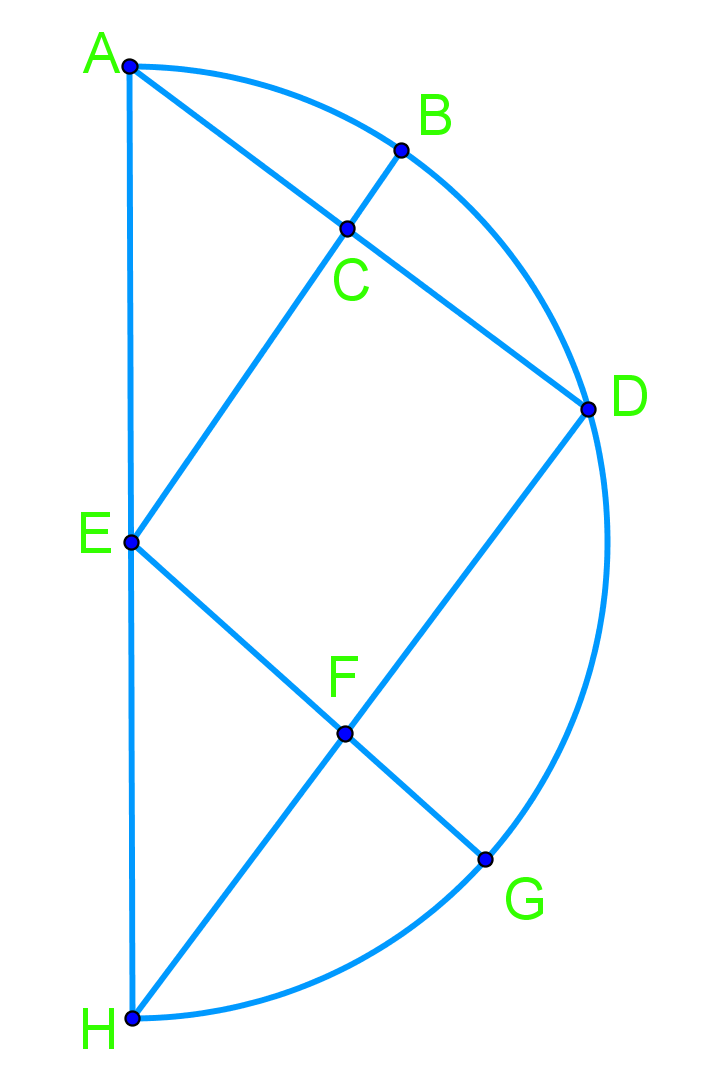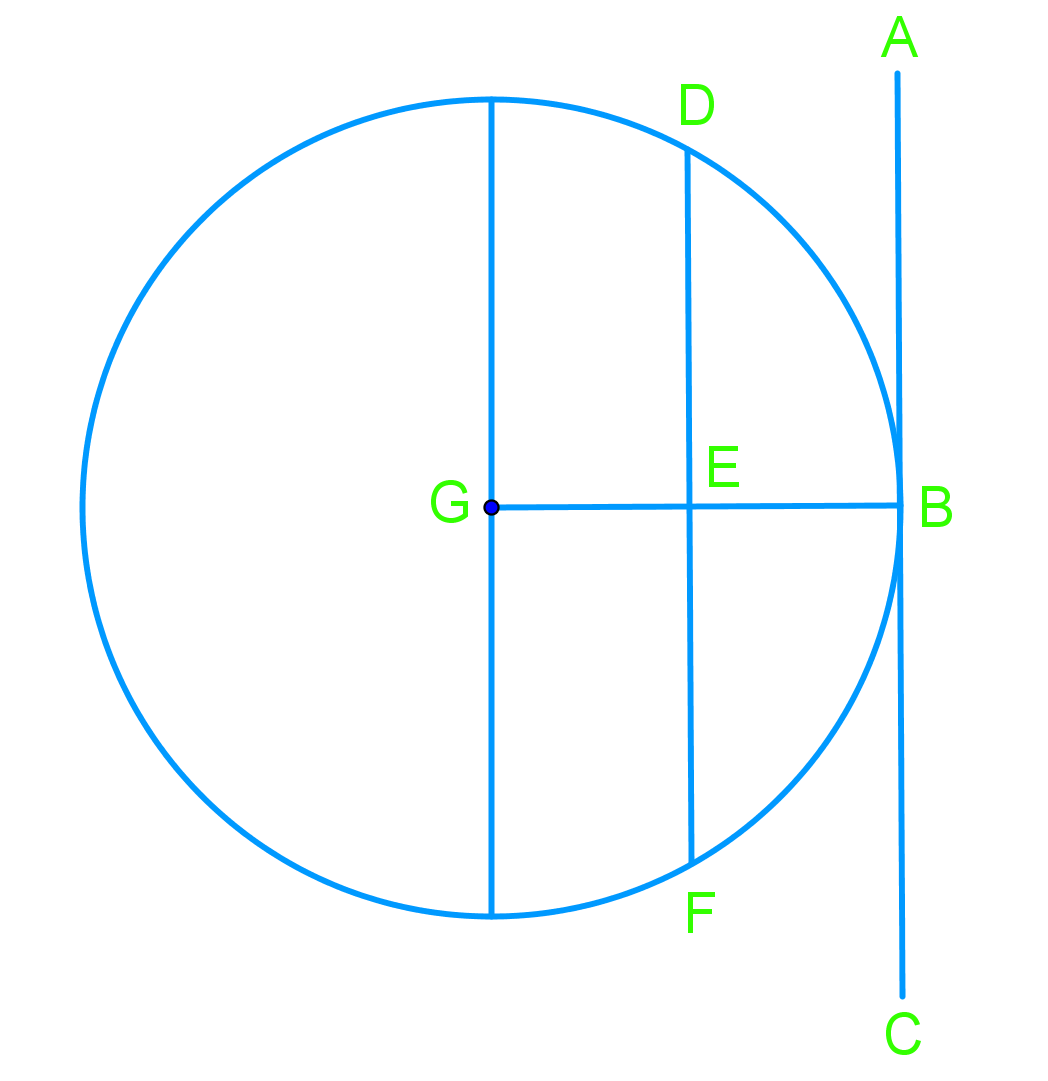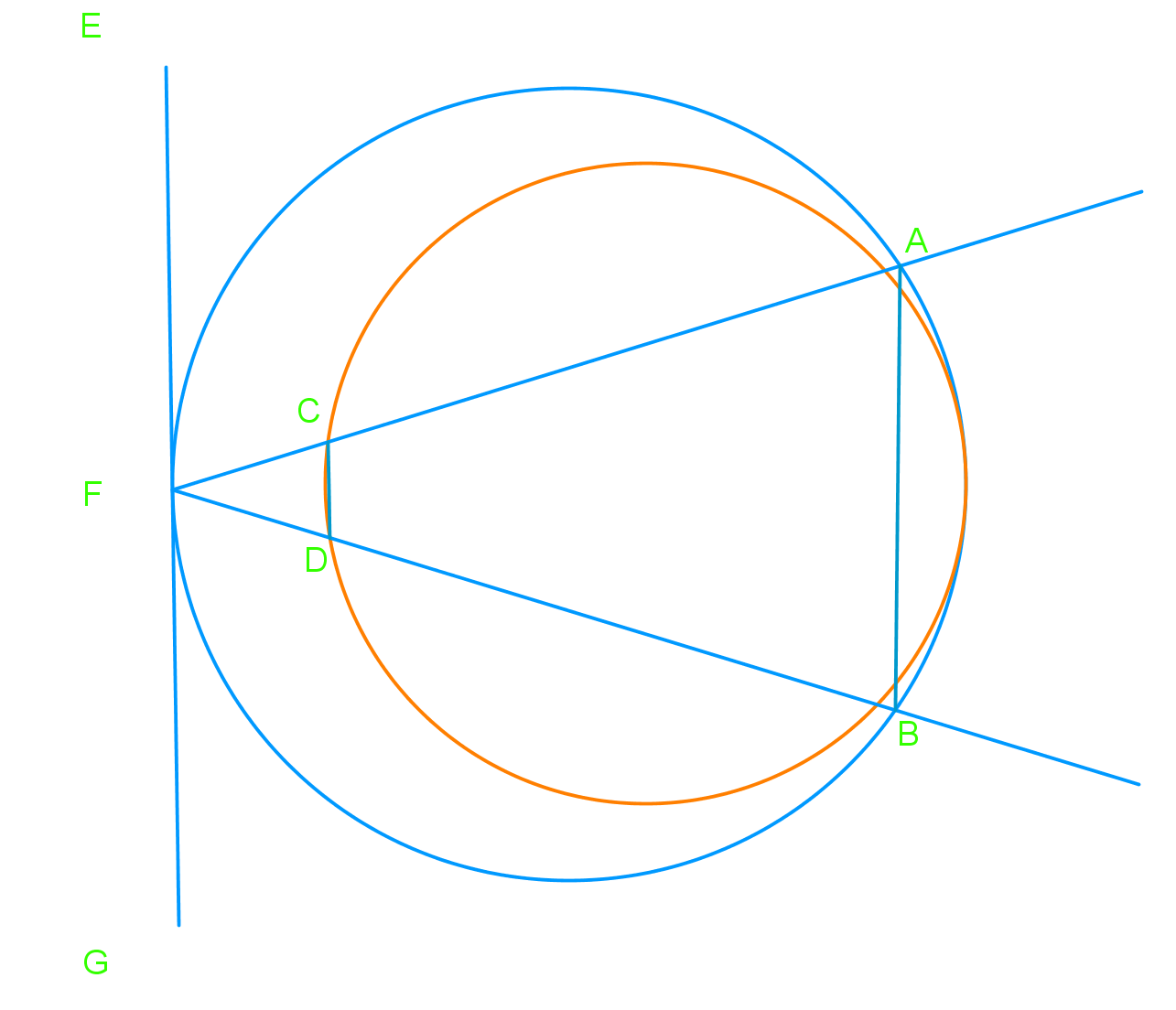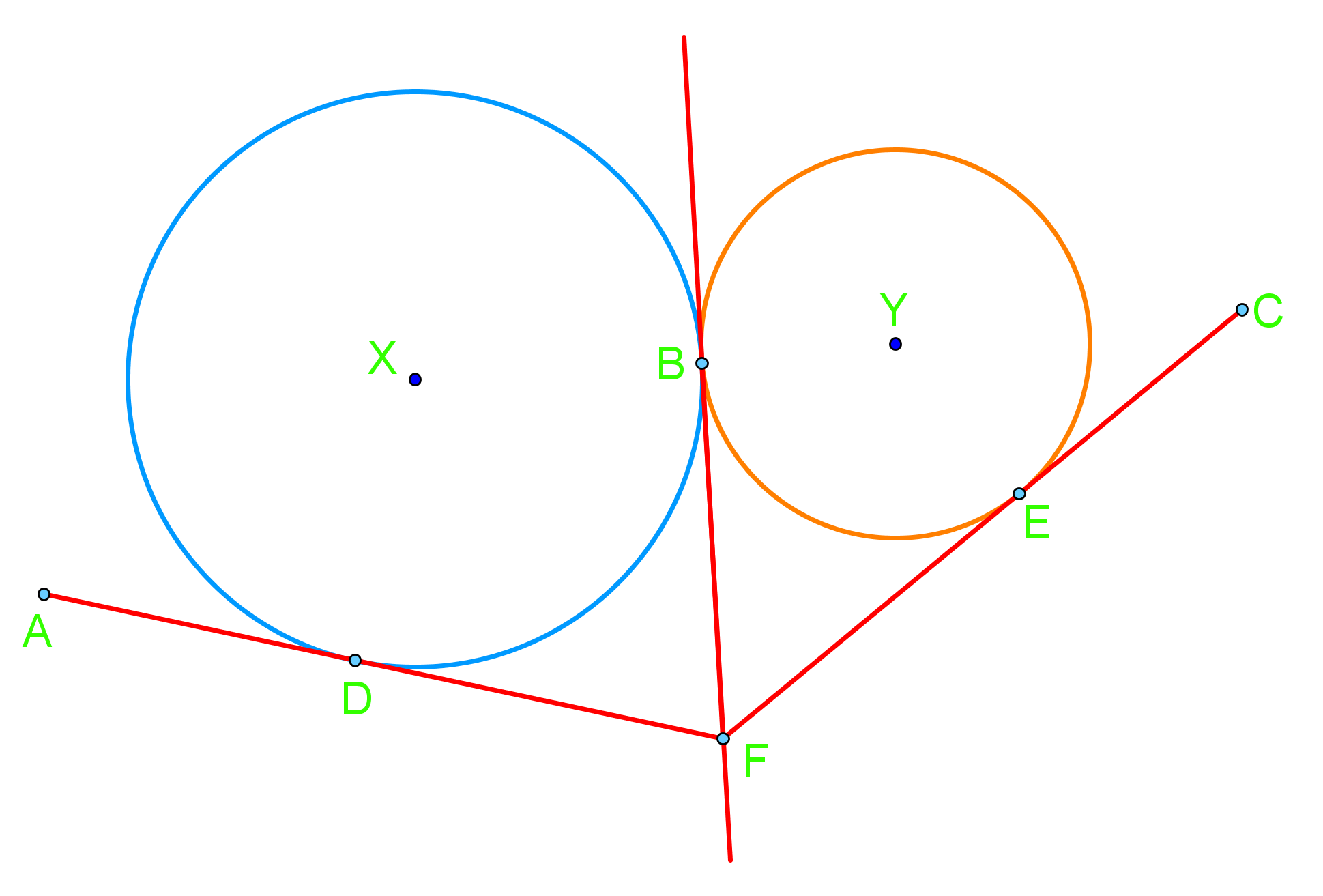# Circle chord, tangent, and inscribed angles proofs

### Circle chord, tangent, and inscribed angles proofs

#### Lessons

• Introduction
a)
1. What is a chord?
2. What is a tangent?

• 1.
Given: 2AC =AD, 2DF=DH Prove: $\angle$BEG = 90°• 2.
Given: AC is tangent at B, AC is parallel to DF Prove: arc BD = arc BF Given: 2AC =AD, 2DF=DH Prove: $\angle$BEG = 90°• 3.
Given: EG is tangent at F, AB is parallel to CD Prove: $\angle$BAF = $\angle$DCF Given: 2AC =AD, 2DF=DH Prove: $\angle$BEG = 90°• 4.
Given: AF is tangent to circle X at D, CF is tangent to circle Y at E. DF tangent to both circles Prove: DF = EF Given: 2AC =AD, 2DF=DH Prove: $\angle$BEG = 90°